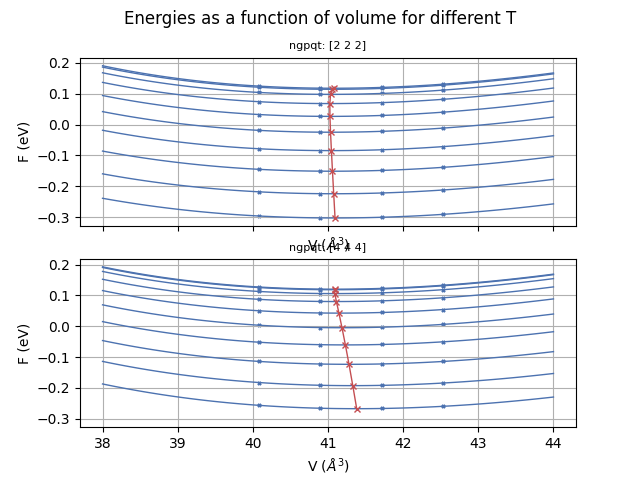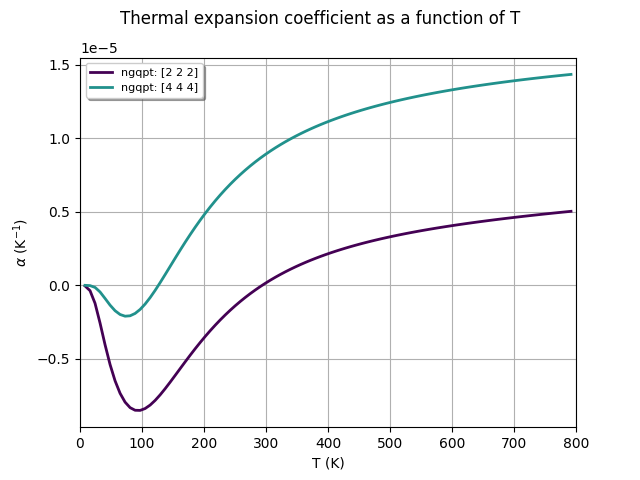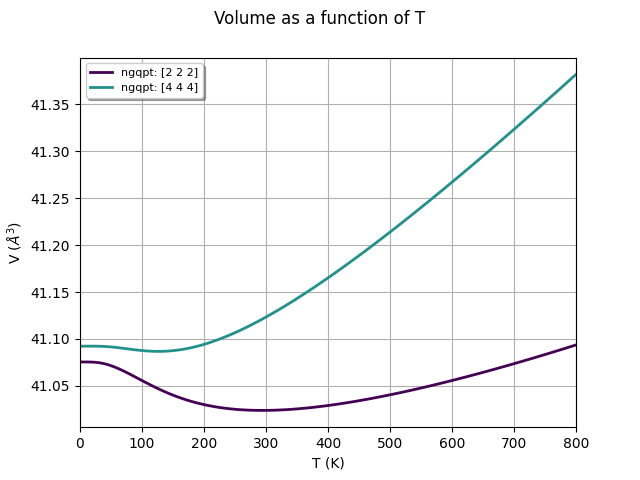# Quasi-harmonic approximation (convergence wrt Q-mesh)¶

This example shows how to use the GSR.nc and PHDOS.nc files computed with different volumes to analyze the convergence of QHA thermodynamic properties with respect to the number of q-points used to compute the phonon DOS.

•••Out:

```<Figure size 640x480 with 1 Axes>
```

```import os
import abipy.data as abidata

from abipy.dfpt.qha import QHAQmeshAnalyzer

# We use a list of GSR.nc and PHDOS.nc files corresponding to different isotropic strains.
# These files are shipped with AbiPy so that we don't need to run calculations from scratch.
#strains = [-4, -2, 0, 2, 4, 6]
strains = [-2, 0, 2, 4]
dirpath = os.path.join(abidata.dirpath, "refs", "si_qha")

gsr_paths = [os.path.join(dirpath, "mp-149_{:+d}_GSR.nc".format(s)) for s in strains]
ddb_paths = [os.path.join(dirpath, "mp-149_{:+d}_DDB".format(s)) for s in strains]

# Initialize QHA object from files.
qhana = QHAQmeshAnalyzer(gsr_paths, ddb_paths)

# To change the default EOS (vinet), use
#qhana.set_eos("vinet")

# Compute ph-DOS with this list of nqsmall values.
qhana.run_qlist([2, 4])

# Analyze convergence. API similar to QHA.
qhana.plot_energies(title="Energies as a function of volume for different T")

qhana.plot_thermal_expansion_coeff(title="Thermal expansion coefficient as a function of T")

qhana.plot_vol_vs_t(title="Volume as a function of T")
```

Total running time of the script: ( 0 minutes 19.360 seconds)

Gallery generated by Sphinx-Gallery